# Finite state machine (FSM) counter design: Gray codes have a useful property in that consecutive numbers differ in only a single bit position. Table 1 lists a 3-bit modulo 8 Gray code representing the... related homework questions

• #### Finite state machine (FSM) counter design: Gray codes have a useful property in that consecutive numbers differ in only a single bit position. Table 1 lists a 3-bit modulo 8 Gray code representing the...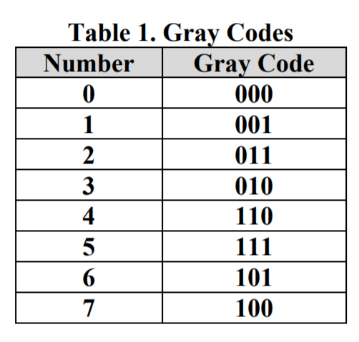Finite state machine (FSM) counter design: Gray codes have a useful property in that consecutive numbers differ in only a single bit position. Table 1 lists a 3-bit modulo 8 Gray code representing the numbers 0 to 7. Design a 3-bit modulo 8 Gray code counter FSM. a) First design and sketch a 3-bit modulo 8 Gray code counter FSM...

• #### It’s review question, I need this as soon as possible. Thank you 3) For thè diferential equation: (a) The point zo =-1 is an ordinary point. Compute the recursion formula for the coefficients of...It’s review question, I need this as soon as possible. Thank you 3) For thè diferential equation: (a) The point zo =-1 is an ordinary point. Compute the recursion formula for the coefficients of the power series solution centered at zo- -1 and use it to compute the first three nonzero terms of the power series when -1)-s and v(-1)-0....

• #### Dont copié formé thé book oh ya dont copié formé thé book cause you Oiil inde up being triste soi remembré not toi copié frome thé book oh ya

Dont copié formé thé book oh ya dont copié formé thé book cause you Oiil inde up being triste soi remembré not toi copié frome thé book oh ya!translation in english please!

• #### Q3. Synchronous Counter Figure 8.3(a) shows a modulo-8 synchronous up-counter (Modulo-8 because this counter can count...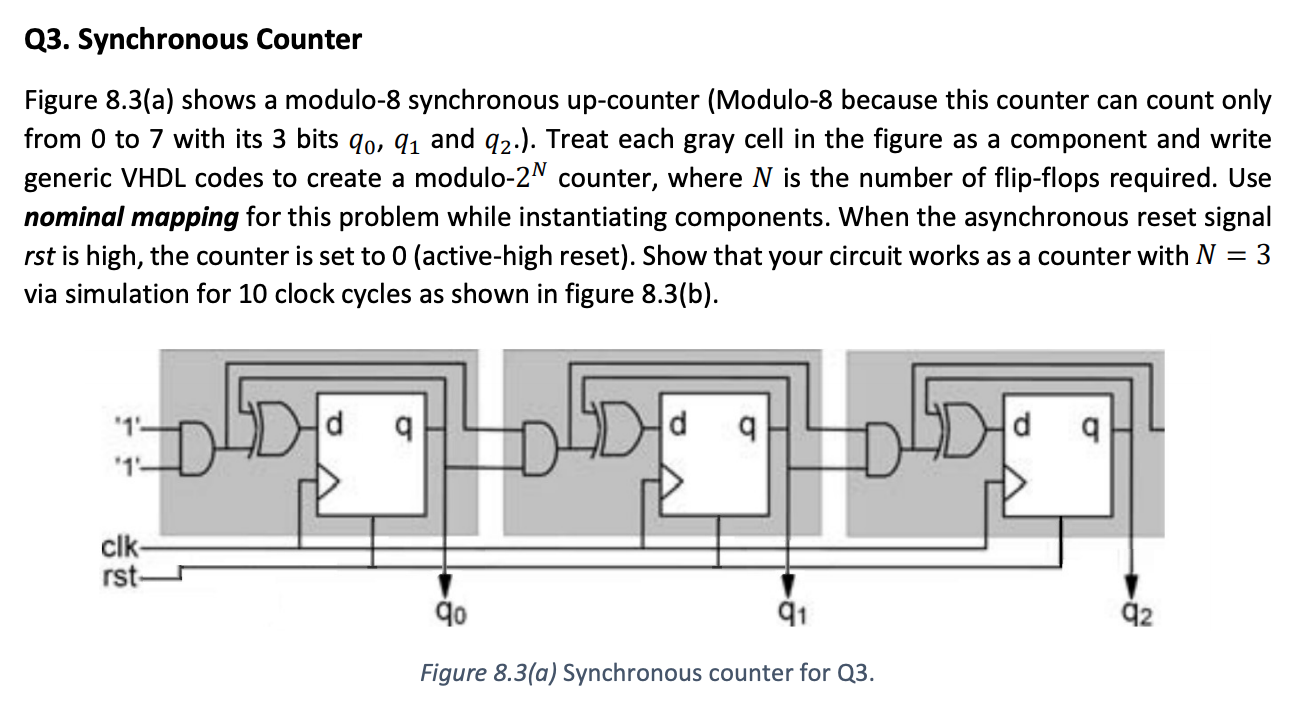Q3. Synchronous Counter Figure 8.3(a) shows a modulo-8 synchronous up-counter (Modulo-8 because this counter can count only from 0 to 7 with its 3 bits qo, q1 and 92.). Treat each gray cell in the figure as a component and write generic VHDL codes to create a modulo-2N counter, where N is the number of flip-flops required. Use nominal mapping...

• #### DSuppose \$39oo is deposited in a savings account that increases exponentially.Detamine thě APv if the acount...DSuppose \$39oo is deposited in a savings account that increases exponentially.Detamine thě APv if the acount increases to \$t020 in 4 years. Ass ume tne interest Vale remains Constant and no additional deposits or Withdrawals are made. (a.) Let pbe the APY. Note tnat if tme inital balaqe is yo, ne year later tne balane is %more. P- 3 (Tpe...

• #### Table Q4.1 shows the state transition table for a finite state machine (FSM) with one input...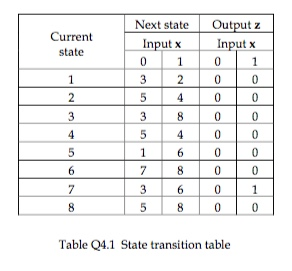Table Q4.1 shows the state transition table for a finite state machine (FSM) with one input x, one output z and eight states. (a) Copy the table of Table Q4.2 into your examination book and determine the states and outputs for the input listed, assuming a start current state of ‘1’. Determine what function the FSM is performing. (b) Using...

• #### Write assembly or C software to implement the following Mealy FSM (Figure 2.42). Include the FSM state machine, port ini...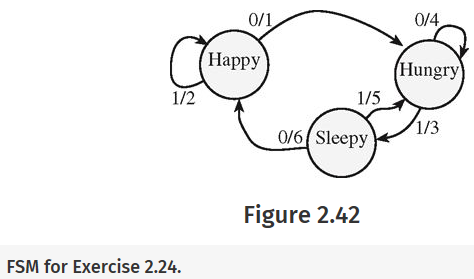Write assembly or C software to implement the following Mealy FSM (Figure 2.42). Include the FSM state machine, port initialization, timer initialization, and the FSM controller. The command sequence will be input, output, wait 10 ms, input, then branch to next state. The 1-bit input is on Port P (PP0), and the 3-bit output is on Port P (PP3, PP2,...

• #### a) A synchronous finite state machine (FSM) is described by the state table in Fig. 3. Show how redundant states may...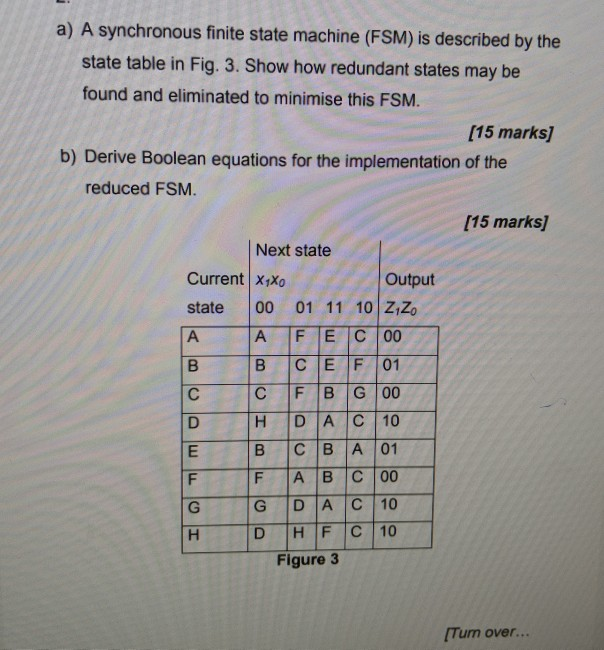a) A synchronous finite state machine (FSM) is described by the state table in Fig. 3. Show how redundant states may be found and eliminated to minimise this FSM. [15 marks) b) Derive Boolean equations for the implementation of the reduced FSM. (15 marks] Next state Output Current X1Xo state 00 01 11 10 Z1Zo A A F E C...

• #### Question 9 [7 Marks] A state table for a finite state machine (FSM) is given below....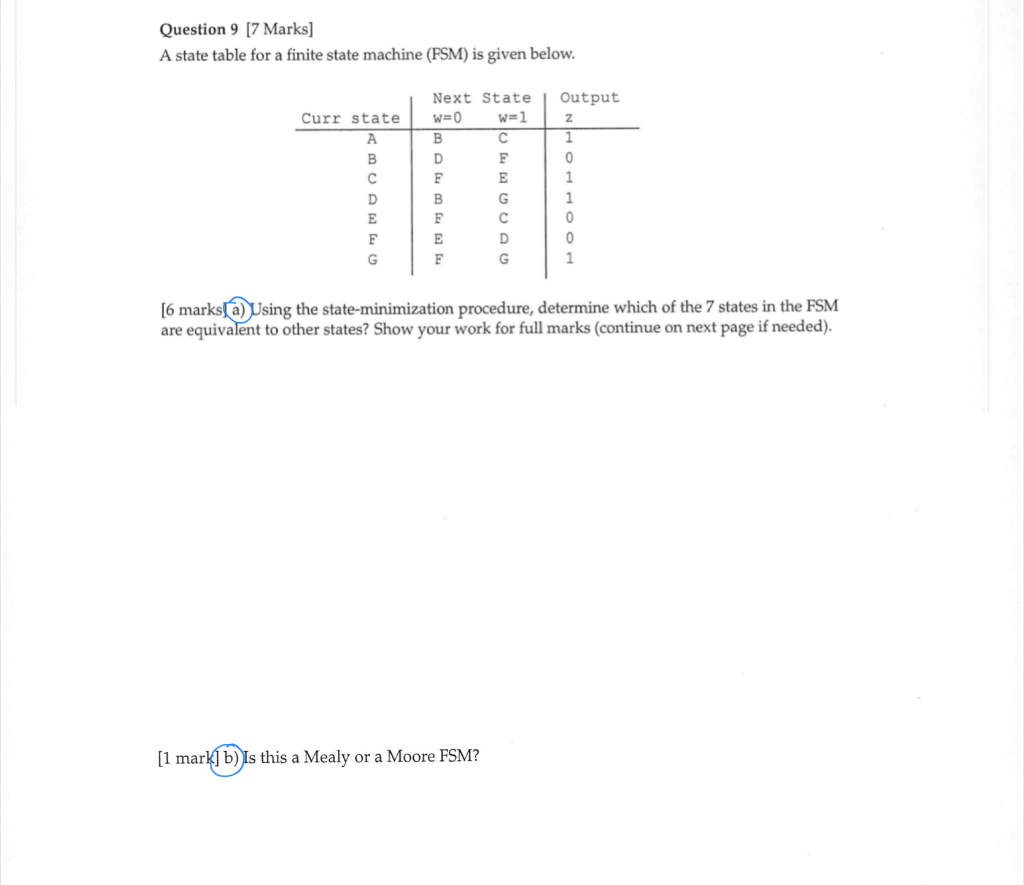Question 9 [7 Marks] A state table for a finite state machine (FSM) is given below. Output Next State w=0 w=1 Curr state 1 [6 marks[a) Using the state-minimization procedure, determine which of the 7 states in the FSM are equivalent to other states? Show your work for full marks (continue on next page if needed). [1 mark] b) Is...

• #### Consider a 4-bit binary counter that increments on every clock pulse. (a) Construct the state diagram for a counter that has an state variable word A3A2A1A0. (b) Construct the state table by assuming...

Consider a 4-bit binary counter that increments on every clock pulse. (a) Construct the state diagram for a counter that has an state variable word A3A2A1A0. (b) Construct the state table by assuming that the circuit consists of four D-type flip-flops with the inputs D3, D2, D1, D0 corresponding to the outputs A3, A2, A1, A0, respectively. (c) Determine the...

• #### 6. Suggest a method for representing rational numbers on a Turing machine, then sketch a method for adding and subtracting such numbers. 6. Suggest a method for representing rational numbers...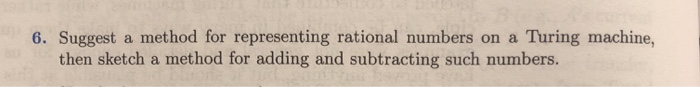6. Suggest a method for representing rational numbers on a Turing machine, then sketch a method for adding and subtracting such numbers. 6. Suggest a method for representing rational numbers on a Turing machine, then sketch a method for adding and subtracting such numbers.

• #### Your supervisor has assigned you to build a finite state machine (FSM) in C code to control the o...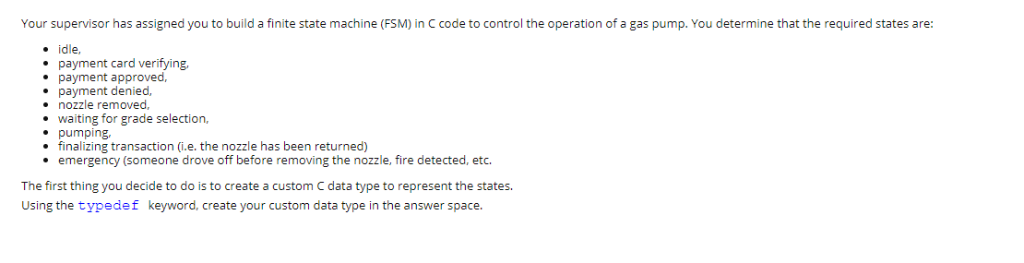Your supervisor has assigned you to build a finite state machine (FSM) in C code to control the operation of a gas pump. You determine that the required states are: . idle, payment card verifying payment approved, payment denied, nozzle removed, waiting for grade selection, e pumping. finalizing transaction (i.e. the nozzle has been returned) . emergency (someone drove off...

• #### 6.(15 points) a)Write VHDL code for the following Soda Dispenser controller. It is a synchronous FSM with an active high Reset signal. b) What type of FSM is? States: Init, Wait, Disp Inputs Enou...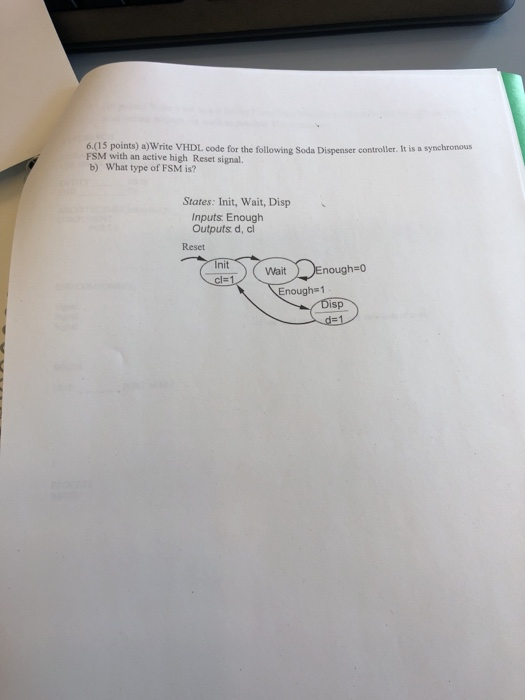6.(15 points) a)Write VHDL code for the following Soda Dispenser controller. It is a synchronous FSM with an active high Reset signal. b) What type of FSM is? States: Init, Wait, Disp Inputs Enough Outputs d, cl Reset Init nough o Wait cl= Enough 1 isp 6.(15 points) a)Write VHDL code for the following Soda Dispenser controller. It is a...

• #### design using mealy or moore FSM Design a system that we can fit in our mobile device so that it will lock the mobile only if we type a wrong pin number 3 consecutive times. show all your work....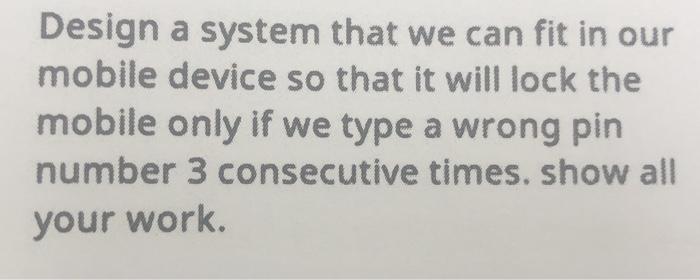design using mealy or moore FSM Design a system that we can fit in our mobile device so that it will lock the mobile only if we type a wrong pin number 3 consecutive times. show all your work. Design a system that we can fit in our mobile device so that it will lock the mobile only if we...

• #### 0/3 D6.15 Write an assembly main program that implements this Mealy finite state machine. happy The FSM state graph...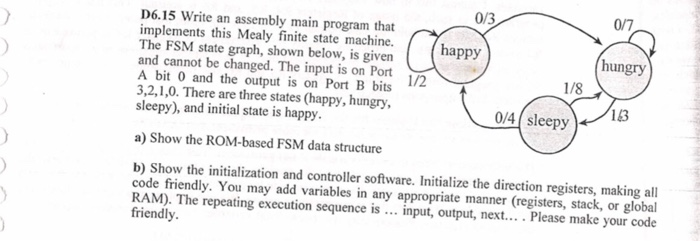0/3 D6.15 Write an assembly main program that implements this Mealy finite state machine. happy The FSM state graph, shown below, is givenP and cannot be changed. The input is on Port A bit 0 and the output is on Port B bits 3,2,1,0. There are three states (happy, hungry, sleepy), and initial state is happy. hungry 1/8 1/2 143...

• #### I am completely confused on understanding the differences between Commutative property Associative property Identity property Inverse property: Multiplication property of zero Distributive property for Addition and Multiplication

I am completely confused on understanding the differences between Commutative property Associative property Identity property Inverse property: Multiplication property of zero Distributive property for Addition and Multiplication. I am given the pictures on ALEKS to see it, but I don't have a written explaination on how to tell the differences when identifying which is which. Is there a simple explaination...

• #### Which of the following codes is used to provide positioning relative to the nearest container? 1. a) position: fixed b) position: static; c) position: absolute d) position: relative; e) position:...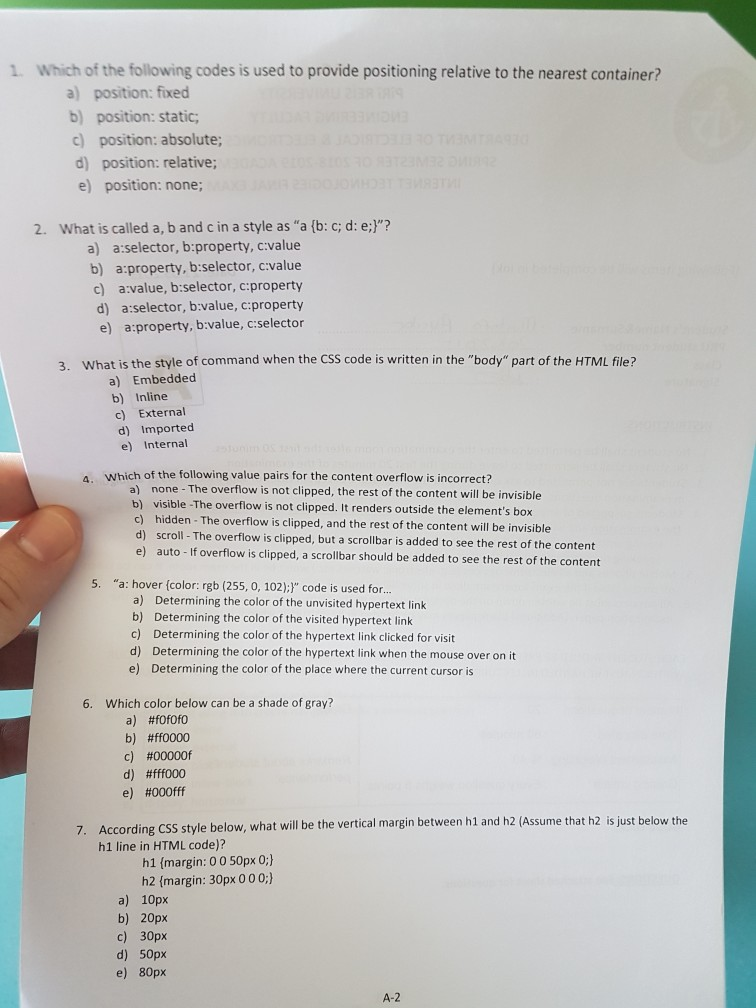Which of the following codes is used to provide positioning relative to the nearest container? 1. a) position: fixed b) position: static; c) position: absolute d) position: relative; e) position: none; 2. What is called a, b and c in a style as "a (b: c; d: e:]"? a) a:selector, b:property, c:value b) a:property, b:selector, cvalue c) avalue, b:selector, c:property...

• #### Please show how this answer was obtained. Problem #6 (15 points) A 12-bit hamming code was generated from an 8-bit code using the format as follows Original 8-bit value Modified 8-bit value (12-bt ham...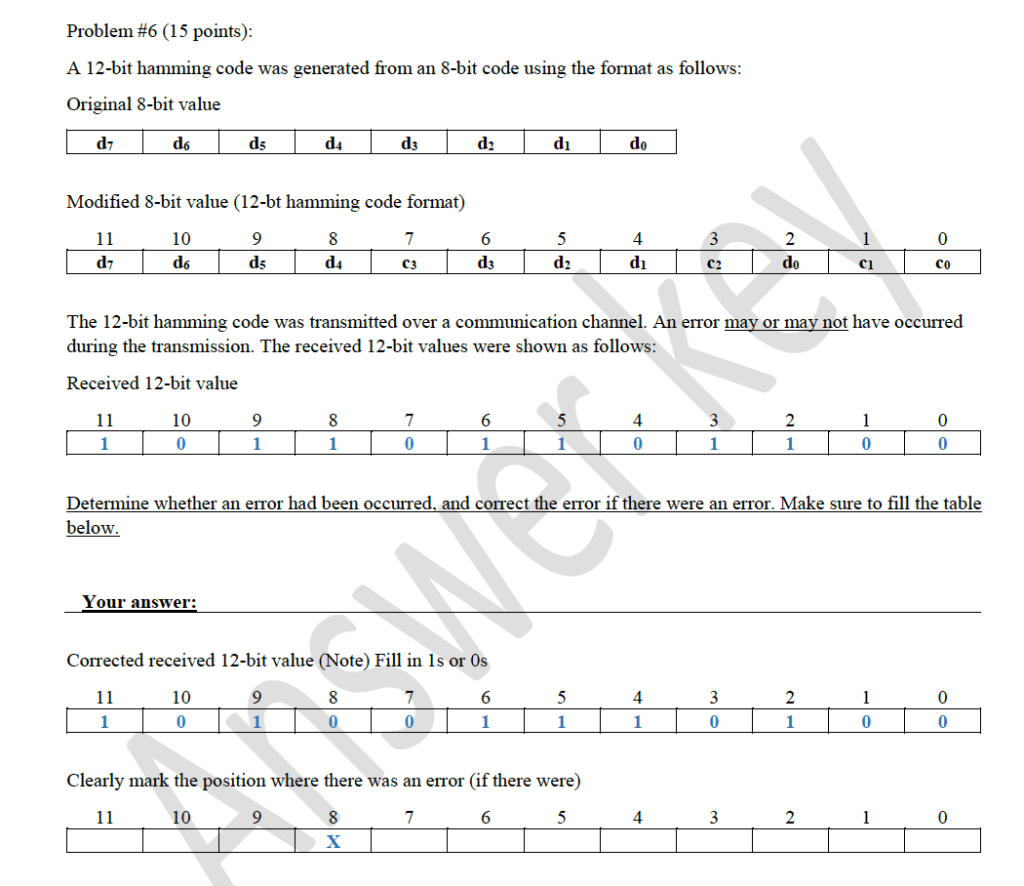Please show how this answer was obtained. Problem #6 (15 points) A 12-bit hamming code was generated from an 8-bit code using the format as follows Original 8-bit value Modified 8-bit value (12-bt hamming code format) 10 9 C3 co The 12-bit hamming code was transmitted over a communication channel. An error may or may not have occurred during the...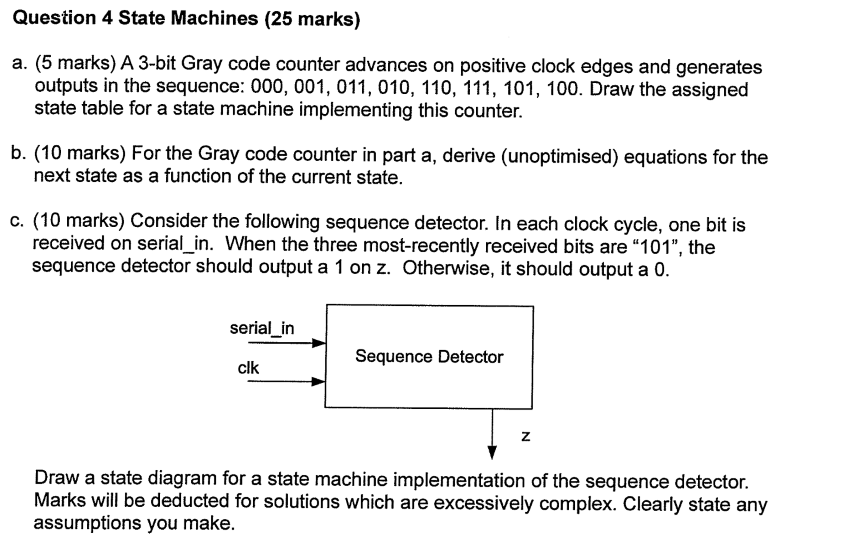Question 4 State Machines (25 marks) a. (5 marks) A 3-bit Gray code counter advances on positive clock edges and generates outputs in the sequence: 000, 001, 011, 010, 110, 111, 101, 100. Draw the assigned state table for a state machine implementing this counter. b. (10 marks) For the Gray code counter in part a, derive (unoptimised) equations for...